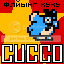-=+=- -=+=- -=+=- -=+=- -=+=- -=+=- -=+=- -=+=- -=+=- -=+=- -=+=- -=+=- -=+=- -=+=- -=+=- -=+=- -=+=- -=+=- -=+=- -=+=- -=+=- -=+=- -=+=- -=+=- -=+=- -=+=- -=+=- -=+=- -=+=- -=+=- (c) WidthPadding Industries 1987 0|501|0 -=+=- -=+=- -=+=- -=+=- -=+=- -=+=- -=+=- -=+=- -=+=- -=+=- -=+=- -=+=- -=+=- -=+=- -=+=- -=+=- -=+=- -=+=- -=+=- -=+=- -=+=- -=+=- -=+=- -=+=- -=+=- -=+=- -=+=- -=+=- -=+=- -=+=-
SoCoder -> Showcase Home -> Simulation

flying_cuccoCreated : 16 September 2008

System : Windows
Language : Cobra

### Dress-up

For WW #168

Dress-up
ScreenshotsA little game where you put clothes on a doll.

Uses OpalGUI and graphics from my RPG. If I get time I'll upload some different clothes from other sets.

### Comments

Tuesday, 16 September 2008, 19:55
blanko1324At first I thought, "Why...why would cucco make a dress-up game."

Then I saw "RPG" and was kind of relieved.

It's good for what it is. Would fit nicely into a RPG.